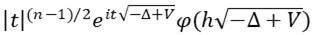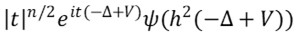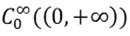# Semi-Classical Dispersive Estimates for the Wave and Schr¨odinger Equations with a Potential in Dimensions ð“ƒ â‰¥ 4

## Abstract

We expand the operatorsand, 0 < h â‰ª 1, modulo operators whose L1 â†’ Lâˆž norm is ON(hN), âˆ€ N â‰¥ 1, where ðœ‘, ðœ“ âˆˆand V âˆˆ Lâˆž(ð“¡ð“ƒ), ð“ƒ â‰¥ 4, is a real-valued potential satisfying V(x) = O (âŒ©xâŒª-ð›¿), ð›¿ > (ð“ƒ + 1)/2 in the case of the wave equation and ð›¿ > (ð“ƒ + 2)/2 in the case of the Schr¨odinger equation. As a consequence, we give sufficent conditions in order that the wave and the Schr¨odinger groups satisfy dispersive estimates with a loss of Î½ derivatives, 0 â‰¤ Î½ â‰¤ (ð“ƒ âˆ’ 3)/2. Roughly speaking, we reduce this problem to estimating the L1 â†’ Lâˆž norms of a finite number of operators with almost explicit kernels. These kernels are completely explicit when 4 â‰¤ ð“ƒ â‰¤ 7 in the case of the wave group, and when ð“ƒ = 4, 5 in the case of the Schr¨odinger group.

## Keywords

• Universidade Federal de Pernambuco, Departamento de Matem´atica, Av. Prof. Luiz Freire, S/N, Cid. Universit´aria, CEP. 50.540-740 – Recife-Pe, Brazil.
• Universit´e de Nantes, D´epartement de Math´ematiques, UMR 6629 du CNRS, 2, rue de la Houssini`ere, BP 92208, 44332 Nantes Cedex 03, France.
• Pages: 01–14
• Date Published: 2008-07-01
• Vol. 10 No. 2 (2008): CUBO, A Mathematical Journal

2008-07-01

## How to Cite


F. Cardoso and G. Vodev, “Semi-Classical Dispersive Estimates for the Wave and Schr¨odinger Equations with a Potential in Dimensions ð“ƒ â‰¥ 4”, CUBO, vol. 10, no. 2, pp. 01–14, Jul. 2008.

Articles